## Sunday, February 24, 2008

### Normalization Techniques in C# 3.0

What do you get from this post?

In this post I would talk elaborately about implementing Normalization Techniques like BCNF Decomposition, 3NF Synthesis and pre-requisites like Test for Losslessness, finding minimal cover of a given set of functional dependencies, etc. I would not be posting the entire source code here, as the intention of this post is to help users get started to write "real" code but not to make their life simple by pasting the code. So the code snippets I paste would be "images" and not text! I apologize for my sadism but I would not like it when my students rip off source code off Google and submit it.

Following this would not only help you learn Normalization techniques but also give you better idea about C# 3.0 features and how to apply them in a real situation. If you are looking at this post, then probably you know C#.

What will I learn?

1. BCNF Decomposition Algorithm
2. 3NF Synthesis
3. How to find if a schema is in BCNF?
4. How to find the minimal cover of the set of functional dependencies?
5. How to find the closure of an FD?
6. Using C# 3.0 Extension Methods
7. Using Object Initializers
8. Using Lambda Expressions in C#
9. Using Linq to write compact code
10. A lot other stuff ....

Did I mention, it took only 540 lines of code to implement both the BCNF Decomposition and 3NF synthesis?

Assumptions

This post assumes you are familiar with what Normalization is. You have a schema R and a set of Functional dependencies, then normalization is a  technique to split the schema R into set of sub-schemas Ri such that each sub schema is in a particular normal form.

Most highly recommended normal forms are the BCNF and 3NF. 3NF is a less strict normal form than the Boyce-Codd Normal form. There are so called the "design algorithms" that researchers have proposed to normalization a given schema.

What is BCNF?

I would not talk about definitions here. If you need elaborate answers then visit this link at Wikipedia. Simply put, a table Ri is said to be NOT in BCNF if there is atleast one functional dependency X->Y that satisfies the schema such that X is not a candidate key. I talked about a relation not being in BCNF because that is what matters during implementation, as you will see later. A positive definition would be : Ri is said to be in BCNF if the Left hand side of all its FDs is a candidate key.

Getting started

Clearly if we are going to implement normal forms, we would need to represent a "Relation", a "Functional Dependency", etc. So the way I organized the system can be seen below. It is a class diagram taken from Visual Studio. Click on the figure below to look at the classes involved.

Relation class

The heart of the implementation is the Relation class. Think properly, how do you define relation mathematically? What interests you about relation? Well, for me, I am bothered with the R and the set of functional dependencies and that is what I have in my relation class.

You can notice that I have a list of Functional Dependencies. I represent each FD as a seperate class called "FunctionalDependency". Assume for now that you have such a class. So what next? You might be interested to implement a closure algorithm, right? But I implemented in FunctionalDependency class. I would talk about the methods when and where required. For now, let us move on to the other important class

FunctionalDependency class

So again, the same question - What do you think should be the state of an object that represents a functional dependency? Well, again my answer is that it should have LHS,RHS(where LHS->RHS is the FD),  an equivalent "R" which is nothing but LHS+RHS. Also it would be good if we have a member that states if this FD violates BCNF or not? We might also use this member for other instances too where it might violate a different condition. So the functional dependency state would like shown below.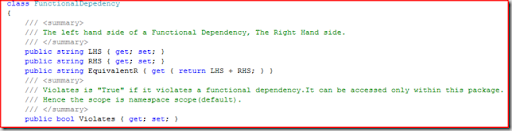So why the hell did I  chose R, LHS,RHS all to be strings? An example of R would be like "ABCDEFGH" where each letter corresponds to an attribute. So instead of working hard with a list or an array, it would be easier to work with strings(we have string#ToCharArray() to our rescue). But this would require us to write a set of methods that would let you perform set like operations on the strings. For example, if you merge two schemas "ABC" and "BCD" you expect to get "ABCD". But a string + would give you "ABCBCD". So we need a method like "ABC".SetAdd("BCD") which would give return "ABCD" instead of "ABCBCD". So here comes the extension methods.

How do we implement extension methods in C#?

The important point to remember is that extension methods should be implemented in static classes only. The methods I implemented that perform "set-operations" on strings are SetAdd(),SetMinus(),SetEquals(). The code implemented makes good use of LINQ extension methods. And since I intend to concentrate on Normalization in this post, I would skip detailed explanation about extension methods.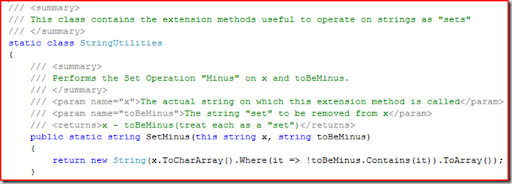Note that the class is static and the method is static. Another point to note that the first parameter is marked as "this string" meaning we wish to make SetMinus() as an extension method to the string class. After this, we could use SetMinus() as shown below.

`string s = "Krishna";string output = s.SetMinus("kna");Console.WriteLine(output);//prints "rish"`

In the above method, I am just taking all the characters in the "x" which are not in the string set that is to be "minus"d. See how sweet LINQ and Lambda expressions are.

The other methods are listed. I hope you understand the statements, if not, drop me an email so that I can write down explanation. Note that all these methods are in the same static class StringUtilities.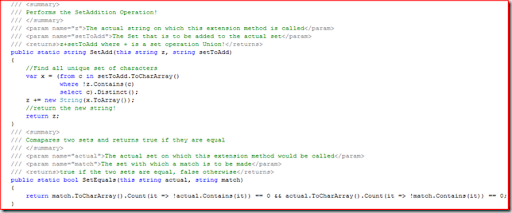The SetAdd() makes use LINQ expressions to find all the unique characters that are in setToAdd and that are not in the actual expression. It also makes clever use of LINQ aggregator method Distinct(). One thing I noticed is that as a beginner developer you would pride in writing programs with more lines of code. But as you mature as a developer you would enjoy writing compact code and these days nothing gives me more satisfaction than writing one liner or two liner to do a good job.

Anyway now that we are set to start, we can now look at how decomposition is implemented. For programing sake, I have a super class called Decomposition which can be seen in the class diagram above. The class listing is shown below.

The static method GetUnpreservedDependencies() is not yet implemented and the idea is to implement it using Armstrong's axioms.

The method IsLossLess() takes in a Decomposition object and returns true if the decomposition is lossless and false otherwise. This is used to test our implementation of both BCNF decomposition and 3NF Sythesis - both of which are theoretically lossless. The Decomposition class defines an abstract method ApplyDecomposition() which should be implemented by the inheriting classes. It returns the list of decomposed schemas which is also the "DecomposedSchemas" property. I know schemas is not a correct word and a plural for schema is just schema, so forgive me. Now let us look at the BCNF Decomposition algorithm.

BCNF Decomposition in C#

The algorithm to implement BCNF Decomposition is

1. Add the current relation (of type Relation) to the list of DecomposedSchemas.

2. Get all the schema that is not in BCNF. Check the Relation for BCNF violation using the method InBCNF(Relation r) which is listed as shown below.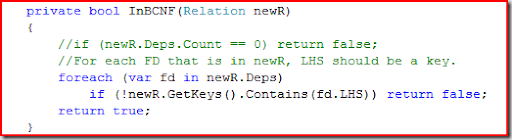3. If there are no such schema that violate BCNF, then you quit.

4. For any schema sch that is not in BCNF, do the following steps.

1. Pick a functional dependency that violates BCNF. There is a method in Relation#GetViolations() that returns a list of violating FunctionalDependencies. Its implementation is shown below.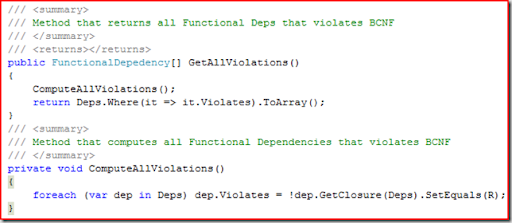A dependency "dep" violates BCNF if its closure does not result in R, the actual schema. The closure is computed using all the Dependencies in the relation. It is defined in the FunctionalDependency class as listed below.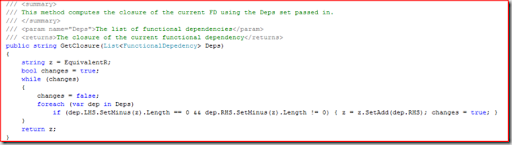The algorithm is taken from the book Database Systems:An Application Oriented Approach

2. Having picked a FD(x->y) that violates BCNF, you now get two relations called r (right) and l (left), where l = FD.EquivalentR (which is x+y) and r = (sch.R - FD.RHS)+FD.LHS (Which is Y). You add both the schema to the DecomposedSchemas collection.

5. Return the list of decomposed schema.

The code listing for the entire BCNF Decomposition is shown below.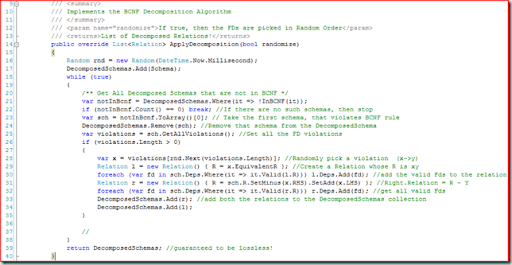Just a recap of what we have seen till now in this post. We have looked at how to compute closure in C#, how to implement the BCNF decomposition algorithm, how to use extension methods, how to use LINQ query to fetch unique characters. For this section, finally we will look at how to test the BCNF Decomposition we implemented. The output you see would be something like this.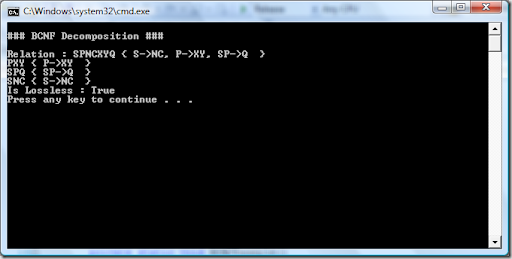The main method is shown below.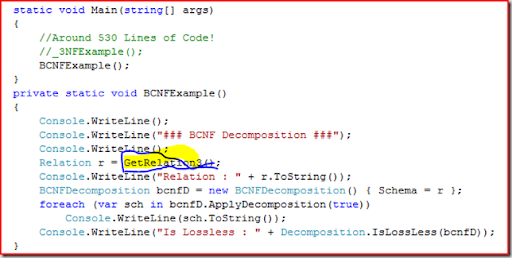You can see a very badly highlighted method GetRelation3() which returns a Relation. The method listing is shown below. This method also shows on how we actually constructed our relation.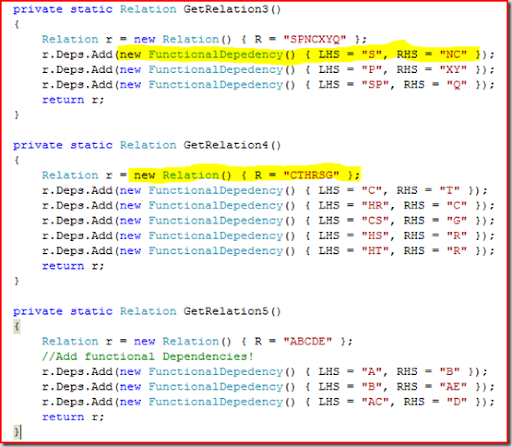In the above code you can see other sample relations too. But look at the syntax for Object initializers. (the one marked in yellow). For both Relation as well as FunctionalDependency creation, we have made use of the beautiful initializers saving us atleast 50 lines of code for all the examples.

So how did we test for losslessness?

Testing for losslessness in decomposition

The key is the theorem which states

"If a closure of Ri gives R, then the decomposition is lossless".

The implementation is simple. For all subschema obtained, we compute its closure using the dependencies of the original schema. It the resultant of the closure gives R for any of the subschema, then the decomposition is lossless. Now you might be confused and might be wondering if you need to write new code! to compute closure for schema. The answer is no! remember you have implemented closure method in the FunctionalDependency class. You can create a new FD whose LHS is Ri and RHS is empty and then call the method GetCLosure(). This works like magic and without any additional code! The complete code is listed below. Notice that we are not bothered if the decomposition is BCNF Decomposition or 3NF synthesis. We can check for any such decomposition for its losslessness nature.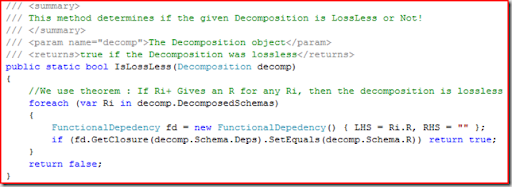Now that we have looked at BCNF Decomposition and also a good C# 3.0 features that enables developers write some good compact code, we would look at 3NF synthesis in the next post. I hope this post is informative enough to get you started. I have shown all the code and with clever reading of Class diagram picture and the snippets you should be able to implement the decomposition algorithm by yourself.

#### 1 comment:Anonymous said...

Krishna, in the "InBCNF(Relation r)" method you call "newR.GetKeys()", I don't see the code for this method anywhere in your post. I assume GetKeys() returns the minimal keys for relation "newR". Is that correct?